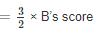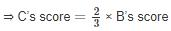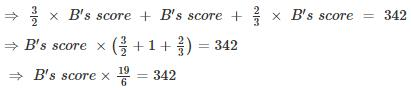Courses

# Three students A, B and C play cricket. The runs scored by A and B respectively are in the ratio 3 : 2. B’s runs to C’s runs are also in the same ratio. Together they score 342 runs. Then the runs scored by B area)216b)108c)90d)171Correct answer is option 'B'. Can you explain this answer? Related Test: Quantitative Aptitude - Test 9

## SSC QuestionPULKIT PANDEY Sep 05, 2019
Given, runs scored by A and B respectively are in the ratio 3 : 2.
∴ A’s scoreAlso B’s runs to C’s runs are also in the same ratio.
∴ B’s score : C’s score = 3 : 2Together they scored 342 runs.
∴ A’s score + B’s score + C’s score = 342⇒ B’s score = 108

This discussion on Three students A, B and C play cricket. The runs scored by A and B respectively are in the ratio 3 : 2. B’s runs to C’s runs are also in the same ratio. Together they score 342 runs. Then the runs scored by B area)216b)108c)90d)171Correct answer is option 'B'. Can you explain this answer? is done on EduRev Study Group by SSC Students. The Questions and Answers of Three students A, B and C play cricket. The runs scored by A and B respectively are in the ratio 3 : 2. B’s runs to C’s runs are also in the same ratio. Together they score 342 runs. Then the runs scored by B area)216b)108c)90d)171Correct answer is option 'B'. Can you explain this answer? are solved by group of students and teacher of SSC, which is also the largest student community of SSC. If the answer is not available please wait for a while and a community member will probably answer this soon. You can study other questions, MCQs, videos and tests for SSC on EduRev and even discuss your questions like Three students A, B and C play cricket. The runs scored by A and B respectively are in the ratio 3 : 2. B’s runs to C’s runs are also in the same ratio. Together they score 342 runs. Then the runs scored by B area)216b)108c)90d)171Correct answer is option 'B'. Can you explain this answer? over here on EduRev! Apart from being the largest SSC community, EduRev has the largest solved Question bank for SSC.

### Related Content

This discussion on Three students A, B and C play cricket. The runs scored by A and B respectively are in the ratio 3 : 2. B’s runs to C’s runs are also in the same ratio. Together they score 342 runs. Then the runs scored by B area)216b)108c)90d)171Correct answer is option 'B'. Can you explain this answer? is done on EduRev Study Group by SSC Students. The Questions and Answers of Three students A, B and C play cricket. The runs scored by A and B respectively are in the ratio 3 : 2. B’s runs to C’s runs are also in the same ratio. Together they score 342 runs. Then the runs scored by B area)216b)108c)90d)171Correct answer is option 'B'. Can you explain this answer? are solved by group of students and teacher of SSC, which is also the largest student community of SSC. If the answer is not available please wait for a while and a community member will probably answer this soon. You can study other questions, MCQs, videos and tests for SSC on EduRev and even discuss your questions like Three students A, B and C play cricket. The runs scored by A and B respectively are in the ratio 3 : 2. B’s runs to C’s runs are also in the same ratio. Together they score 342 runs. Then the runs scored by B area)216b)108c)90d)171Correct answer is option 'B'. Can you explain this answer? over here on EduRev! Apart from being the largest SSC community, EduRev has the largest solved Question bank for SSC.